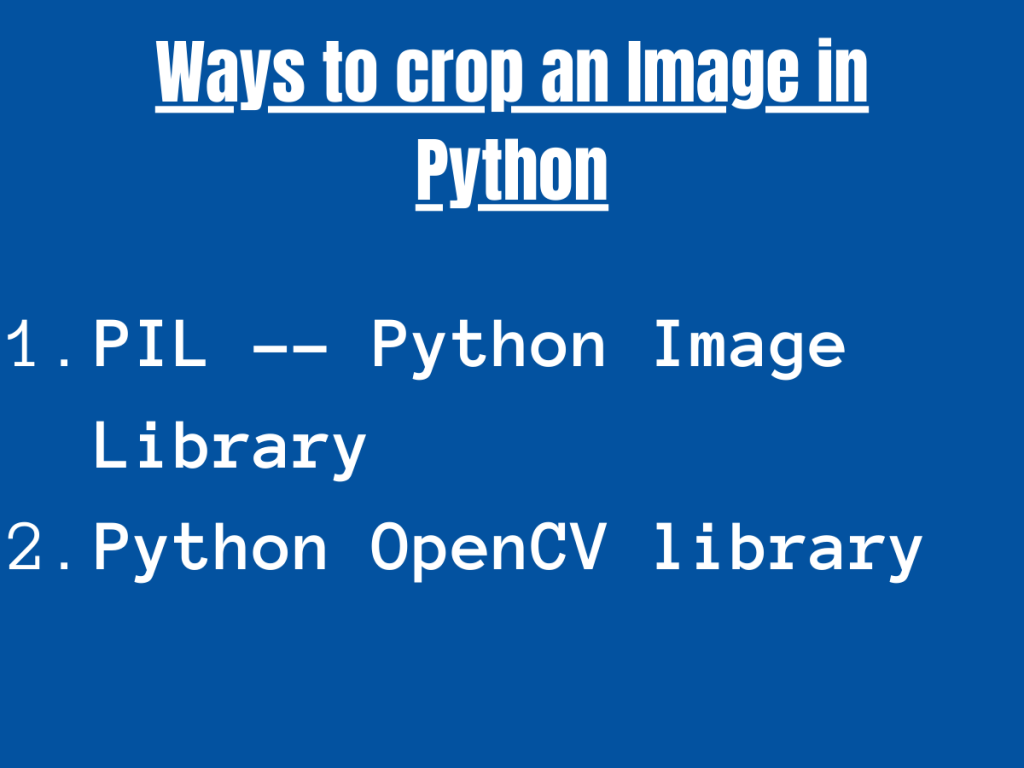# How to crop an image in PythonHello! In this article, we will be focusing on different ways to crop an image in Python. Now, let us unveil and understand the background functions being used to crop an image.

## Technique 1: Python PIL to crop an image

PIL stands for ‘Python Image Library‘. PIL adds image editing and formatting features to the python interpreter. Thus, it has many in-built functions for image manipulation and graphical analysis.

PIL has in-built `Image.crop() function` that crops a rectangular part of the image.

Syntax:

```Image.crop(left, top, right, bottom)
```
• `top `and `left`: These parameters represent the top left coordinates i.e (x,y) = (left, top).
• `bottom `and `right`: These parameters represent the bottom right coordinates i.e. (x,y) = (right, bottom).

The area to be cropped is represented as follows:

• left <= x < right
• top <= y < bottom

Example:

```from PIL import Image

img = Image.open(r"C:\Users\HP\OneDrive\Desktop\<image>.png")

left = 0
top = 50
right = 510
bottom = 292

img_res = img.crop((left, top, right, bottom))

img_res.show()

```

In the above example, `Image.open(r"image path")` is a function of PIL to open an image in read mode.

We have assigned certain values to the left, right, top and bottom coordinates.

`Image.show()` function is used to display the cropped image.

Original Image:

Cropped Image (output):

## Technique 2: Crop an Image in Python using OpenCV

Python OpenCV is a library with a large number of functions available for real-time computer vision. It contains a good set of functions to deal with image processing and manipulation of the same.

In order to process an image using OpenCV, the users need to install OpenCV library with a version of 3.0 and above.

At first, we need to import the OpenCV library in our program using the below code snippet:

```import cv2
```

OpenCV actually performs slicing of the image passed as an array in the method of cropping an image.

Syntax:

```image[start_x:end_x, start_y:end_y]
```
• The image[] actually slices the image in the form of arrays by passing the start and end index of x and y coordinates.
• Thus, the image between the start and end coordinates of x and y is returned as the cropped array object.

Example:

```import cv2

y=0
x=0
h=300
w=510
crop_image = image[x:w, y:h]
cv2.imshow("Cropped", crop_image)
cv2.waitKey(0)
```

The `cv2.imread(r"image path")` function is used to open an image in read mode.

Further, the start and end indexes for the x and y-axis are provided and thus the image is cropped.

The `cv2.imshow()` function is used to display the cropped image. We’ve used the same image as before here.

Cropped Image (output):